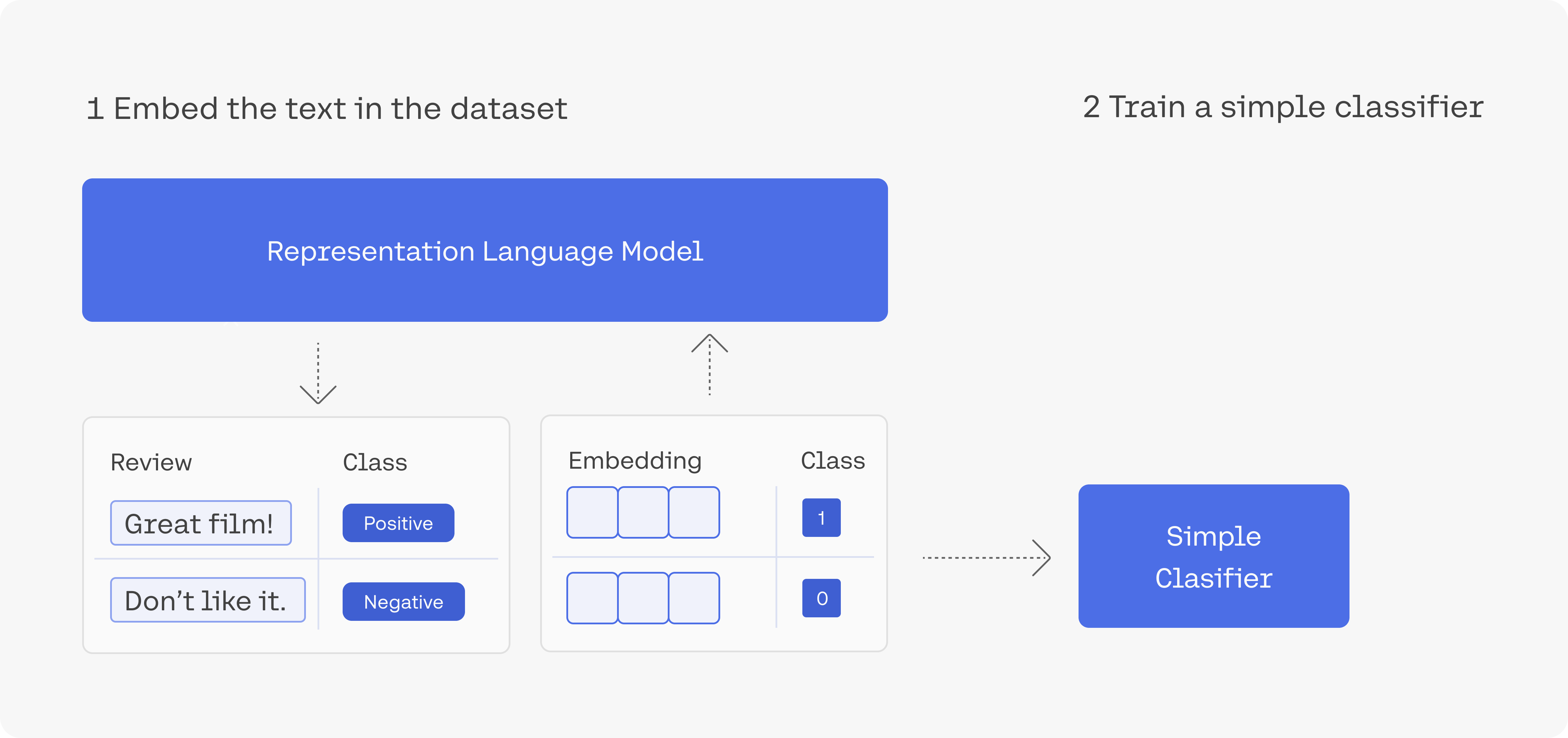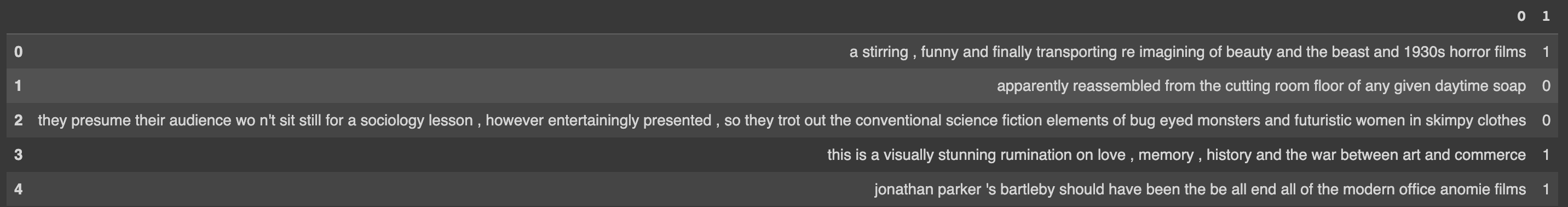# Text Classification (Embed)

📘

This Guide Uses the Embed Endpoint.

This notebook shows how to build a classifiers using Cohere's embeddings. You can find the code on Github or in this colab notebook.First we embed the text in the dataset, then we use that to train a classifier.

The example classification task here will be sentiment analysis of film reviews. We'll train a simple classifier to detect whether a film review is negative (class 0) or positive (class 1).

We'll go through the following steps:

1. Install Cohere
2. Get the dataset
3. Get the embeddings of the reviews (for both the training set and the test set)
4. Train a classifier using the training set
5. Evaluate the performance of the classifier on the testing set

## 1. Install Cohere and Other Dependencies

``````pip install cohere scikit-learn
``````

If you're running an older version of the SDK you'll want to upgrade it, like this:

``````pip install --upgrade cohere
``````

## 2. Get the Dataset

``````import cohere
from sklearn.model_selection import train_test_split

import pandas as pd
pd.set_option('display.max_colwidth', None)

import requests

# Get the SST2 training and test sets
``````

### 2a. Print an Example from the Dataset

``````# Let's glance at the dataset
``````We'll only use a subset of the training and testing datasets in this example. We'll only use 500 examples since this is a toy example. You'll want to increase the number to get better performance and evaluation.

The train_test_split method splits arrays or matrices into random train and test subsets.

``````# Set the number of examples from the dataset
num_examples = 500
# Create a dataframe that
df_sample = df.sample(num_examples)

# Split into training and testing sets
sentences_train, sentences_test, labels_train, labels_test = train_test_split(
list(df_sample), list(df_sample), test_size=0.25, random_state=0)

# The embeddings endpoint can take up to 96 texts, so we'll have to truncate
# sentences_train, sentences_test, labels_train, and labels_test.

sentences_train = sentences_train[:95]
sentences_test = sentences_test[:95]

labels_train = labels_train[:95]
labels_test = labels_test[:95]
``````

## 2a. Set up the Cohere client to embed your reviews

``````# Add the model name, API key, URL, etc.
model_name = "embed-english-v3.0"
api_key = ""

# Here, we're setting up the data objects we'll pass to the embeds endpoint.
input_type = "classification"

# Create and retrieve a Cohere API key from dashboard.cohere.ai
co = cohere.Client(api_key)
``````

## 2b. Use Co.embed() to embed your test and training set

We are calling the co.embed() method to convert our text examples into numerical representations.

``````# Embed the training set
embeddings_train = co.embed(texts=sentences_train,
model=model_name,
input_type=input_type
).embeddings

# Embed the testing set
embeddings_test = co.embed(texts=sentences_test,
model=model_name,
input_type=input_type
).embeddings

# Here we are using the endpoint co.embed()
``````

Note that the ordering of the arguments is important. If you put `input_type` in before `model_name`, you'll get an error.

We now have two sets of embeddings; `embeddings_train` contains the embeddings of the training sentences, while `embeddings_test` contains the embeddings of the testing sentences.

Curious what an embedding looks like? We can print one out:

``````print(f"Review text: {sentences_train}")
print(f"Embedding vector: {embeddings_train[:10]}")
``````

The results look something like this:

``````Review text: the movie 's major and most devastating flaw is its reliance on formula , though , and it 's quite enough to lessen the overall impact the movie could have had
Embedding vector: [3.1484375, 0.56884766, 1.2861328, 0.83154297, 1.5849609, 0.037872314, 1.2617188, 0.40039062, -0.36889648, 0.8671875]
``````

## 3. Train a Classifier Using the Training Set

Now that we have the embedding we can train our classifier using an SVM from sklearn. We call the make_pipeline, which configures a pipeline. The purpose of the pipeline is to assemble several steps that can be cross-validated together while setting different parameters.

``````# import SVM classifier code
from sklearn.svm import SVC
from sklearn.pipeline import make_pipeline
from sklearn.preprocessing import StandardScaler

# Initialize a support vector machine, with class_weight='balanced' because
# our training set has roughly an equal amount of positive and negative
# sentiment sentences
svm_classifier = make_pipeline(StandardScaler(), SVC(class_weight='balanced'))

# fit the support vector machine
svm_classifier.fit(embeddings_train, labels_train)

``````

## 4. Evaluate the Performance of the Classifier on The Testing Set

``````# get the score from the test set, and print it out to screen!
score = svm_classifier.score(embeddings_test, labels_test)
print(f"Validation accuracy on Large is {100*score}%!")
``````

Validation accuracy is 88.8%, though you may get a slightly different number when you run this code.

This was a small scale example, meant as a proof of concept and designed to illustrate how you can build a custom classifier quickly using a small amount of labelled data and Cohere's embeddings. If you want to achieve better performance on this task, increase the number of training examples.